# GMAT Math : Calculating whether right triangles are congruent

## Example Questions

### Example Question #34 : Triangles

A right triangle has a height ofand a base of.  In order for another triangle to be congruent, what must be the length of its hypotenuse?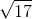Explanation:

In order for two triangles to be congruent, they must be identical. That is, the lengths of the corresponding sides of two congruent triangles must be equal. This means that in order for a triangle to be congruent to one with a height ofand a base of,  its hypotenuse must be the same length as the hypotenuse of that triangle, which we can find using the Pythagorean Theorem: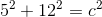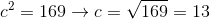### Example Question #35 : Triangles

A given right triangle has a base ofand a height of. What must the base length of a congruent right triangle be?

None of the above.Explanation:

In order for two right triangles to be congruent, the bases and heights must have identical lengths. Since we have a given right triangle with a base of, the congruent right triangle must also have a base of.

### Example Question #36 : Triangles

A given right triangle has a height ofand an acute angle of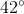. What must the acute angle of a congruent right triangle be?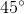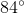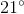None of the above.Explanation:

In order for two right triangles to be congruent, the hypotenuses and acute angles must be identical. Since we have a given right triangle with an acute angle of, the congruent right triangle must also have an acute angle of.

### Example Question #37 : Triangles

A given right triangle has a base ofand a height of. What must the base length of a congruent right triangle be?None of the above.In order for two right triangles to be congruent, the bases and heights must have identical lengths. Since we have a given right triangle with a base of, the congruent right triangle must also have a base of.